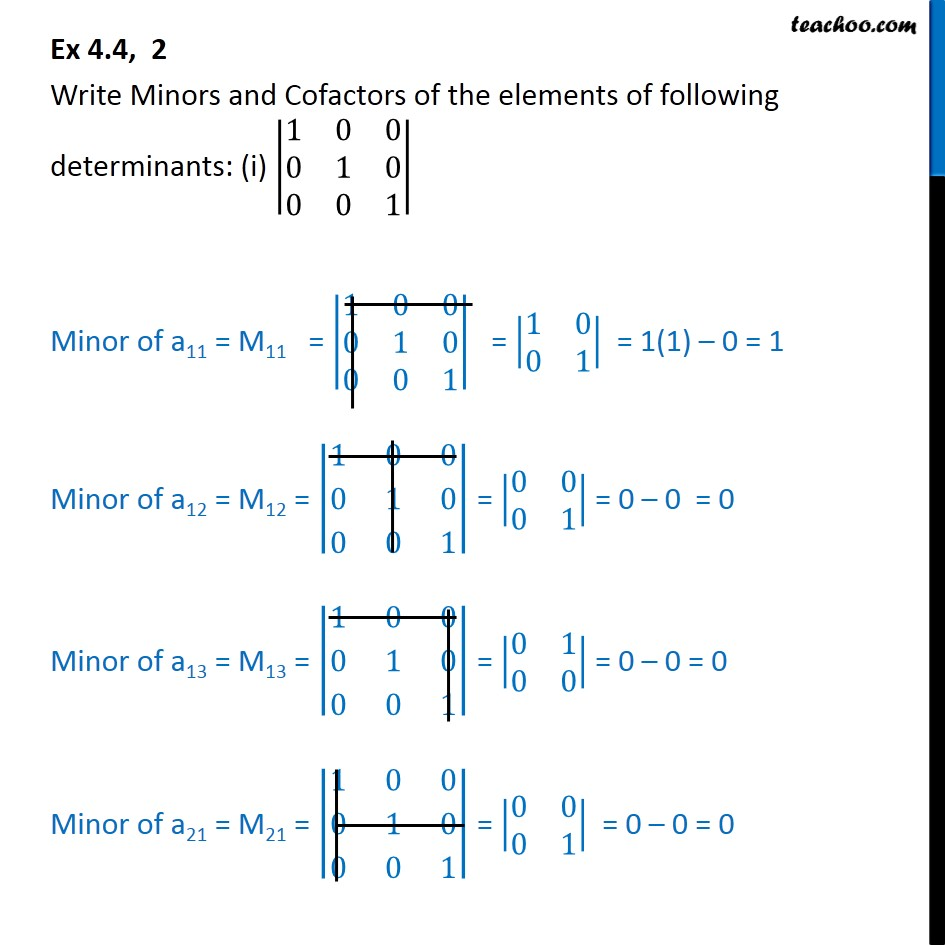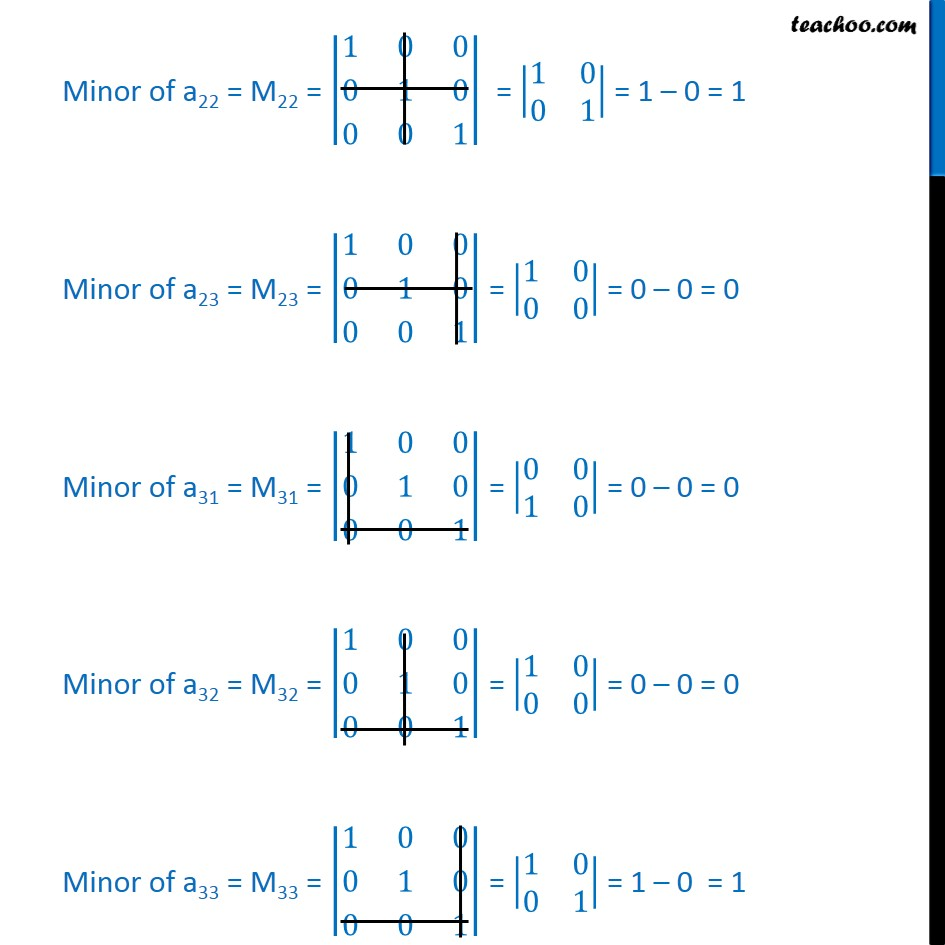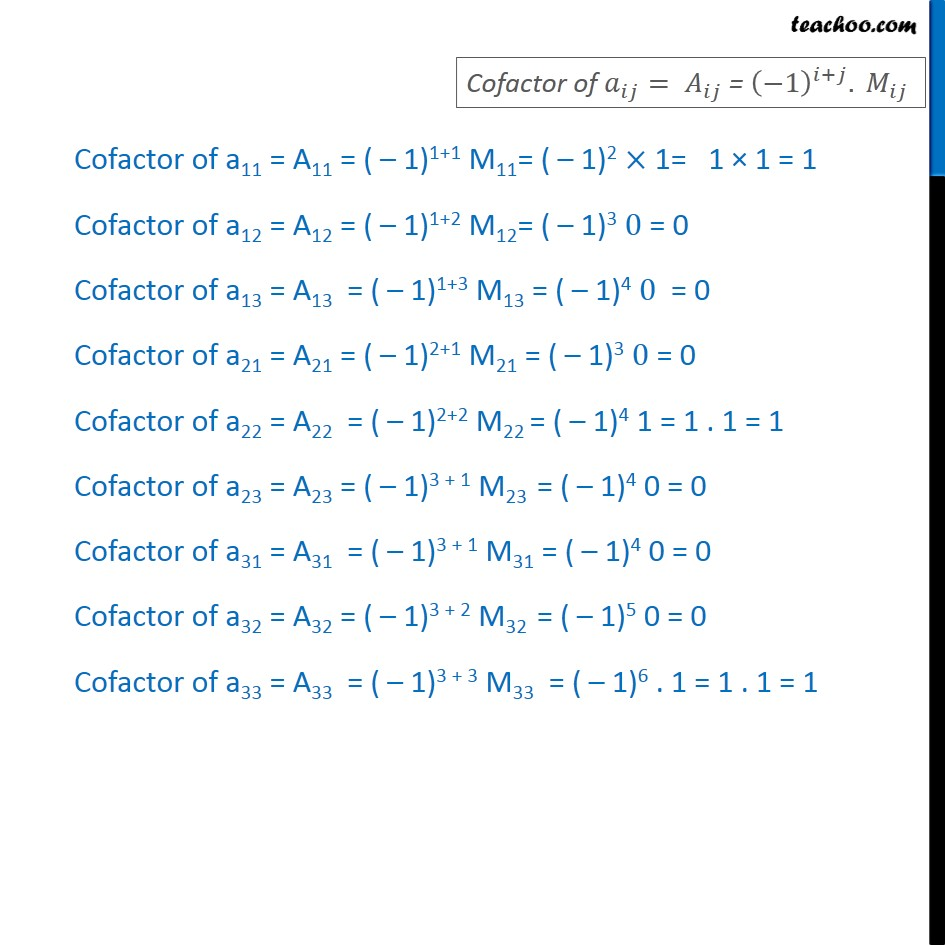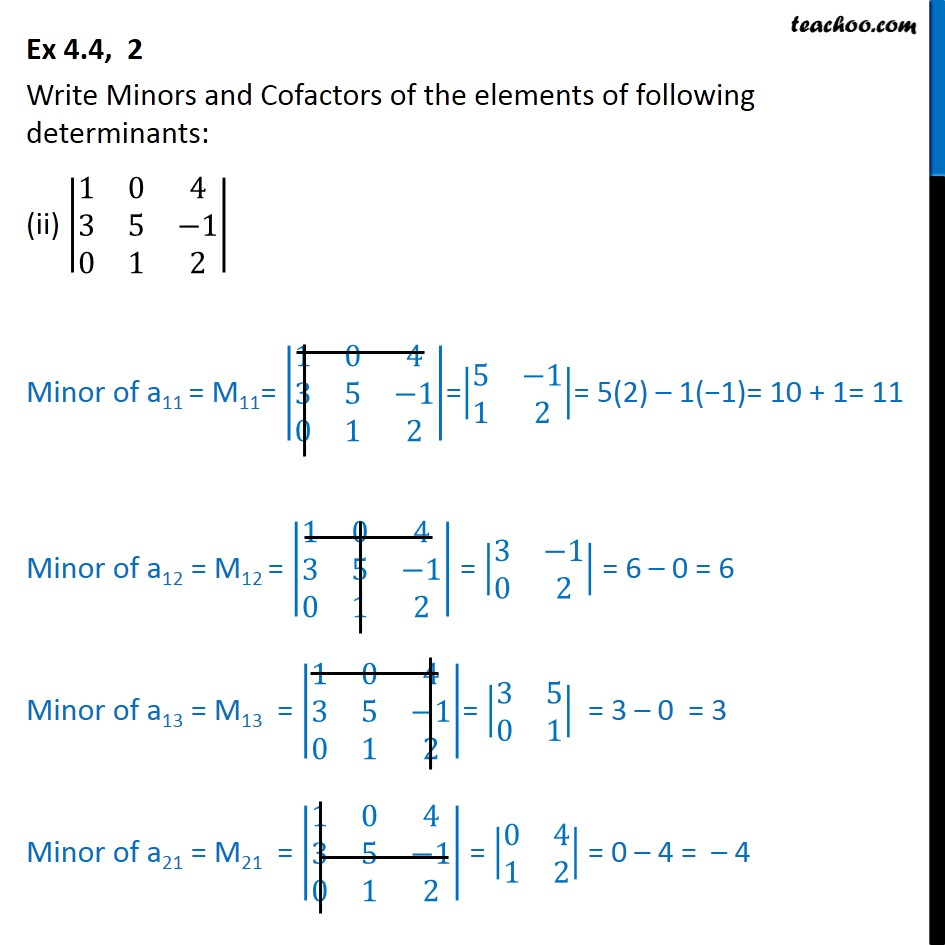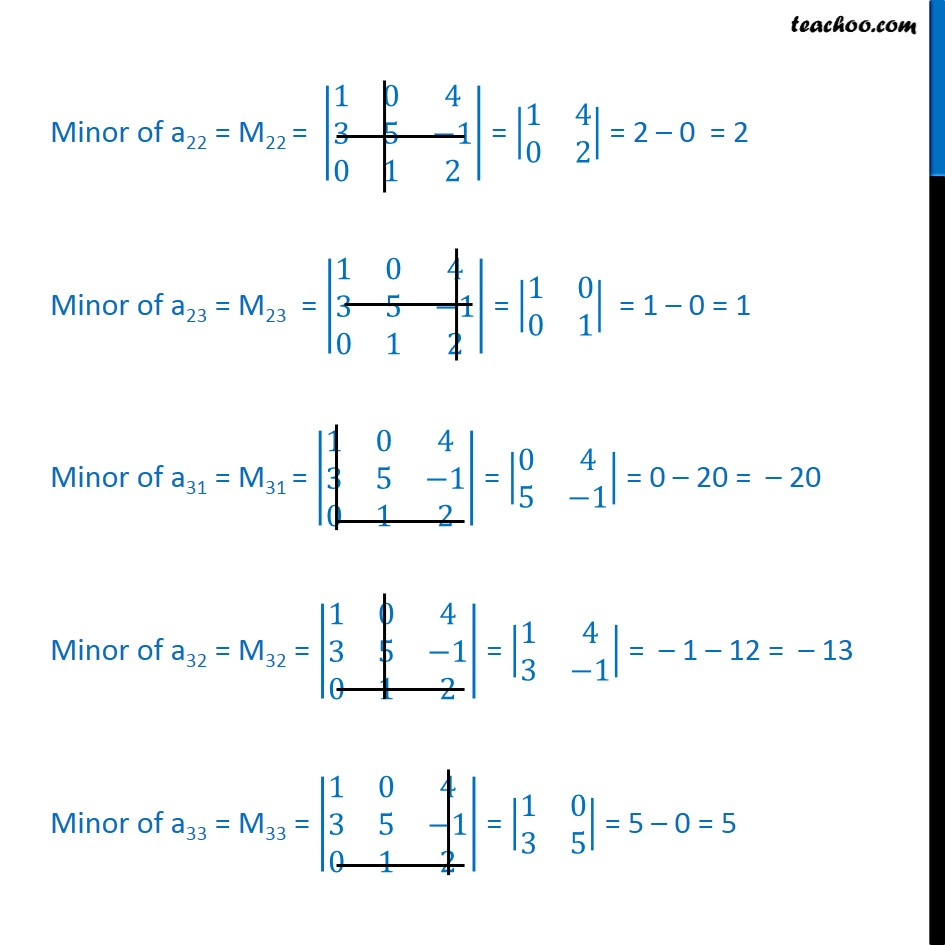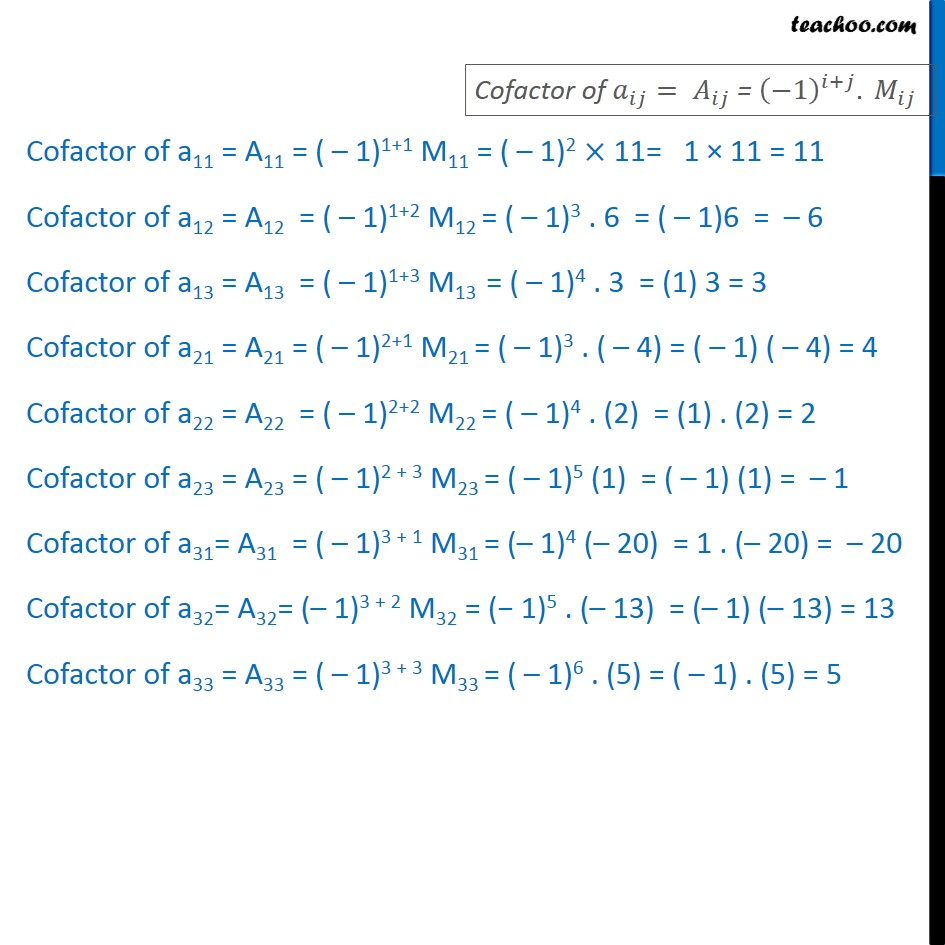Subscribe to our Youtube Channel - https://you.tube/teachoo

1. Chapter 4 Class 12 Determinants
2. Serial order wise
3. Ex 4.4

Transcript

Ex 4.4, 2 Write Minors and Cofactors of the elements of determinants: (i) |■8(1&0&0@0&1&0@0&0&1)| Minor of a11 = M11 = |■8(1&0&0@0&1&0@0&0&1)| = |■8(1&0@0&1)| = 1(1) – 0 = 1 Minor of a12 = M12 = |■8(1&0&0@0&1&0@0&0&1)| = |■8(0&0@0&1)| = 0 – 0 = 0 Minor of a13 = M13 = |■8(1&0&0@0&1&0@0&0&1)| = |■8(0&1@0&0)| = 0 – 0 = 0 Minor of a21 = M21 = |■8(1&0&0@0&1&0@0&0&1)| = |■8(0&0@0&1)| = 0 – 0 = 0 Minor of a22 = M22 = |■8(1&0&0@0&1&0@0&0&1)| = |■8(1&0@0&1)| = 1 – 0 = 1 Minor of a23 = M23 = |■8(1&0&0@0&1&0@0&0&1)| = |■8(1&0@0&0)| = 0 – 0 = 0 Minor of a31 = M31 = |■8(1&0&0@0&1&0@0&0&1)| = |■8(0&0@1&0)| = 0 – 0 = 0 Minor of a32 = M32 = |■8(1&0&0@0&1&0@0&0&1)| = |■8(1&0@0&0)| = 0 – 0 = 0 Minor of a33 = M33 = |■8(1&0&0@0&1&0@0&0&1)| = |■8(1&0@0&1)| = 1 – 0 = 1 Cofactor of 𝑎_𝑖𝑗= 𝐴_𝑖𝑗 = (−1)^(𝑖+𝑗). 𝑀_𝑖𝑗 Cofactor of a11 = A11 = (–1)1+1 M11= (–1)2 × 1= 1 × 1 = 1 Cofactor of a12 = A12 = (–1)1+2 M12= (–1)3 0 = 0 Cofactor of a13 = A13 = (–1)1+3 M13 = (–1)4 0 = 0 Cofactor of a21 = A21 = (–1)2+1 M21 = (–1)3 0 = 0 Cofactor of a22 = A22 = (–1)2+2 M22 = (–1)4 1 = 1 . 1 = 1 Cofactor of a23 = A23 = (–1)3 + 1 M23 = (–1)4 0 = 0 Cofactor of a31 = A31 = (–1)3 + 1 M31 = (–1)4 0 = 0 Cofactor of a32 = A32 = (–1)3 + 2 M32 = (–1)5 0 = 0 Cofactor of a33 = A33 = (–1)3 + 3 M33 = (–1)6 . 1 = 1 . 1 = 1 Ex 4.4, 2 Write Minors and Cofactors of the elements of determinants: (ii) |■8(1&0&4@3&5&−1@0&1&2)| Minor of a11 = M11= |■8(1&0&4@3&5&−1@0&1&2)|=|■8(5&−1@1&2)|= 5(2) – 1(−1) = 10 + 1 = 11 Minor of a12 = M12 =|■8(1&0&4@3&5&−1@0&1&2)| = |■8(3&−1@0&2)| = 6 – 0 = 6 Minor of a13 = M13 = |■8(1&0&4@3&5&−1@0&1&2)| = |■8(3&5@0&1)| = 3 – 0 = 3 Minor of a21 = M21 = |■8(1&0&4@3&5&−1@0&1&2)| = |■8(0&4@1&2)| = 0 – 4 = –4 Minor of a22 = M22 = |■8(1&0&4@3&5&−1@0&1&2)| = |■8(1&4@0&2)| = 2 – 0 = 2 Minor of a23 = M23 = |■8(1&0&4@3&5&−1@0&1&2)| = |■8(1&0@0&1)| = 1 – 0 = 1 Minor of a31 = M31 = |■8(1&0&4@3&5&−1@0&1&2)| = |■8(0&4@5&−1)| = 0 – 20 = – 20 Minor of a32 = M32 = |■8(1&0&4@3&5&−1@0&1&2)| = |■8(1&4@3&−1)| = –1 – 12 = −13 Minor of a33 = M33 = |■8(1&0&4@3&5&−1@0&1&2)| = |■8(1&0@3&5)| = 5 – 0 = 5 Cofactor of 𝑎_𝑖𝑗= 𝐴_𝑖𝑗 = (−1)^(𝑖+𝑗). 𝑀_𝑖𝑗 Cofactor of a11 = A11 = (–1)1+1 M11 = (–1)2 × 11= 1 × 11 = 11 Cofactor of a12 = A12 = (–1)1+2 M12 = (–1)3 . 6 = (–1)6 = –6 Cofactor of a13 = A13 = (–1)1+3 M13 = (–1)4 . 3 = (1) 3 = 3 Cofactor of a21 = A21 = (–1)2+1 M21 = (–1)3 . (–4) = (–1) (–4) = 4 Cofactor of a22 = A22 = (–1)2+2 M22 = (–1)4 . (2) = (1) . (2) = 2 Cofactor of a23 = A23 = (–1)2 + 3 M23 = (–1)5 (1) = (–1) (1) = –1 Cofactor of a31= A31 = (–1)3 + 1 M31 = (–1)4 (– 20) = 1 . (–20) = – 20 Cofactor of a32= A32= (–1)3 + 2 M32 = (−1)5 . (–13) = (–1) (–13) = 13 Cofactor of a33 = A33 = (–1)3 + 3 M33 = (–1)6 . (5) = (–1) . (5) = 5

Ex 4.4